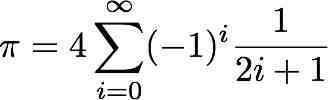# Homework #2

 Due: January 27, 2012 Points: 100

For this homework, please put refinements for all your programs into one file called “refinements.txt” or “refinements.pdf”, and error journal entries for all your programs into one file called “errors.txt” or “errors.pdf”.

The first two problems use the following relationship:1. (30 points) Write a program that approximates the value of π by summing the first n terms of the above series. The program is to prompt the user for n, and then output the sum of the first n terms of this series. (If n is 1, output the value of the first term only.) Your program is also to print the absolute value of the difference between the approximation and the value of math.pi to see how accurate the approximation is.

What your program should show: Suppose the user wants to use 3456 terms. Then your program’s execution should look like this (what the computer prints is in typewriter font, what the user types is in italics style, and ↵ represents typing “enter” or “return”:

How many terms should be used to approximate pi? 3456
After 3456 terms, approximate pi = 3.141303; error is 0.000289

2. (30 points) Now change your program to output the number of terms needed to approximate π to 10−6. Your output should be in the same form as problem 1, except that the number of terms replaces “3456”, and the error should be no more than 0.000001. Your program will not need any input for this problem.

What your program should show: There is no input. The output is to be like the last line of output for problem 1.

3. (40 points) Write a turtle program (see Chapter 3 of the text) to draw an n sided polygon, where the user inputs n. The length of each side of the polygon should be 500/n. The turtle should not be visible at the end.
Hint: At the end of each side, have your turtle turn 360/n degrees. Also, use the method “hideturtle()” to make the turtle invisible.

What your program should show: Suppose the user wants to draw a hexagon (a 6-sided polygon). Then your program’s execution should look like this:

How many sides do you want on the polygon? 6
and then the program draws the hexagon in a new window.ECS 10, Basic Concepts of Computing Winter Quarter 2012